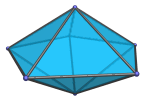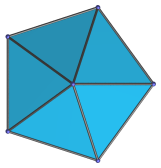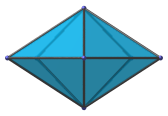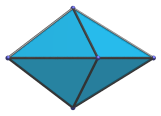# The Pentagonal Bipyramid

The pentagonal bipyramid is the 13th Johnson solid (J13). It has 7 vertices, 15 edges, and 10 faces (10 equilateral triangles).The pentagonal bipyramid can be constructed by attaching two pentagonal pyramids to each other at their bases.

## Projections

Here are some views of the pentagonal bipyramid from various angles:

Projection Envelope DescriptionRegular pentagon

Top view.Rhombus

Side view.Tetragon

Front view.

## Coordinates

The Cartesian coordinates of the pentagonal bipyramid with edge length 2 are:

• (0, 0, ±√((10−2√5)/5))
• (√((10+2√5)/5), 0, 0)
• (√((5−√5)/10), ±φ, 0)
• (−√((5+2√5)/5), ±1, 0)

where φ=(1+√5)/2 is the Golden Ratio.

## Occurrences

The pentagonal bipyramid occurs as cells in some CRF polychora, including (but not limited to):

Last updated 18 Jun 2019.